﻿ Data logger - Netduino Plus 2 (and Netduino Plus 1) - Netduino Forums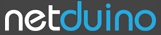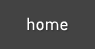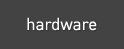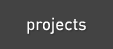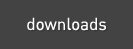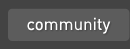The Netduino forums have been replaced by new forums at community.wildernesslabs.co. This site has been preserved for archival purposes only and the ability to make new accounts or posts has been turned off.# Data logger

12 replies to this topic

### #1Zeus

Zeus
• LocationIsrael

Posted 10 April 2012 - 08:58 AM

Hello,

I am trying to write some sensor data on SD card in a csv file.
But in the file I don't see all collected values, rather the last (just one set of three values).
Please correct.

```using System;
using System.Net;
using System.Net.Sockets;
using System.Threading;
using Microsoft.SPOT;
using Microsoft.SPOT.Hardware;
using SecretLabs.NETMF.Hardware;
using SecretLabs.NETMF.Hardware.NetduinoPlus;
using System.IO;
using System.Text;

namespace Integration
{
public class Program
{
private const int MaximumValue = 1023;
private const int AnalogReference = 5;

public static void Main()
{
AnalogInput no2Sensor = new AnalogInput(Pins.GPIO_PIN_A1);
AnalogInput coSensor = new AnalogInput(Pins.GPIO_PIN_A2);
AnalogInput o3Sensor = new AnalogInput(Pins.GPIO_PIN_A3);

while (true)
{
int digitalValue1 = no2Sensor.Read();
float analogValue1 = (float)digitalValue1 / MaximumValue * AnalogReference;

int digitalValue2 = coSensor.Read();
float analogValue2 = (float)digitalValue2 / MaximumValue * AnalogReference;

int digitalValue3 = o3Sensor.Read();
float analogValue3 = (float)digitalValue3 / MaximumValue * AnalogReference;
Debug.Print("NO2 value:\t" + analogValue1.ToString() + "\nCO Value:\t" + analogValue2.ToString() + "\nO3 Value:\t" + analogValue3.ToString());

using (StreamWriter sw = new StreamWriter(@"SD\file.csv"))
{
sw.Write(analogValue1.ToString() + "," + analogValue2.ToString() + "," + analogValue3.ToString());
sw.Write("\n");
sw.Flush();
sw.Close();
}

Thread.Sleep(1000);
}

}

}
}
```

Thanks.

Regards,

Z

### #2Paul Csiki

Paul Csiki
• LocationDublin, Ireland

Posted 10 April 2012 - 09:43 AM

Hello, What is the output of the Debug.Print? Do all three values show up? Also I don't recommend you to create / dispose StreamWriters like that, it's better to leave one active, write data to it, flush, then close it, on the next loop you open, write, flush, close (repeat). Normally everything should work if analogValue1,2,3 have correct values. Cheers.

### #3CW2

CW2

Advanced Member

• Members
•• 1592 posts
• LocationCzech Republic

Posted 10 April 2012 - 10:25 AM

PaulCsiki is right, you should leave the stream open (and preferably flush it after each write). Because every time you create a new instance of StreamWriter class, the file is overwritten if it exists.

### #4Gorf

Gorf

Posted 10 April 2012 - 12:52 PM

My code from my solar inverter log routine:
```if (!File.Exists(LogFileName))
{
using (StreamWriter writer = new StreamWriter(LogFileName, true))
{
writer.WriteLine("Time\tInverter\tTotal today (kWh)\tTotal this month (kWh)\tTotal since install (kWh)\tTotal this year (kWh)\tOutput now (W)");
}
}

strTemp = DateTime.Now.TimeOfDay.ToString();
strTemp = strTemp.Substring(0, strTemp.IndexOf("."));
using (StreamWriter writer = new StreamWriter(LogFileName, true))
{
writer.WriteLine(strTemp + "\t" + InverterData);
}```

I've not bothered logging the date - that's in the filename, and LogfFileName is changed every day.

### #5Paul Csiki

Paul Csiki
• LocationDublin, Ireland

Posted 11 April 2012 - 10:13 AM

Hello,

Here is how I would do it.

```using System;
using System.Net;
using System.Net.Sockets;
using System.Threading;
using Microsoft.SPOT;
using Microsoft.SPOT.Hardware;
using SecretLabs.NETMF.Hardware;
using SecretLabs.NETMF.Hardware.NetduinoPlus;
using System.IO;
using System.Text;

namespace Integration
{
public class Program
{
private const int MaximumValue = 1023;
private const int AnalogReference = 5;
private static StreamWriter sw;

public static void Main()
{
AnalogInput no2Sensor = new AnalogInput(Pins.GPIO_PIN_A1);
AnalogInput coSensor = new AnalogInput(Pins.GPIO_PIN_A2);
AnalogInput o3Sensor = new AnalogInput(Pins.GPIO_PIN_A3);
sw = new StreamWriter(@"SD\file.csv");

while (true)
{
try
{
int digitalValue1 = no2Sensor.Read();
float analogValue1 = (float)digitalValue1 / MaximumValue * AnalogReference;

int digitalValue2 = coSensor.Read();
float analogValue2 = (float)digitalValue2 / MaximumValue * AnalogReference;

int digitalValue3 = o3Sensor.Read();
float analogValue3 = (float)digitalValue3 / MaximumValue * AnalogReference;
Debug.Print("NO2 value:\t" + analogValue1.ToString() + "\nCO Value:\t" + analogValue2.ToString() + "\nO3 Value:\t" + analogValue3.ToString());

sw.Write(analogValue1.ToString() + "," + analogValue2.ToString() + "," + analogValue3.ToString());
sw.Write("\n");
sw.Flush();

Thread.Sleep(1000);
}
catch (Exception)
{
sw.Close();
sw.Dispose();
break;
}
}
}
}
}```

### #6Zeus

Zeus
• LocationIsrael

Posted 11 April 2012 - 11:10 PM

First of all, sorry for late reply. Second, thanks all and especially Paul. Tomorrow I will check the code and update in here. Best, Z

### #7Zeus

Zeus
• LocationIsrael

Posted 12 April 2012 - 02:55 PM

Yes! This code works for logging data unto SD card. I also have an RTC - DS1307, I need to log the time in the CSV file corresponding to analog pin values. I have set time of RTC already using Arduino. With Netduino I only want to read and log time. Please help. Thanks. Best, Z

### #8Paul Csiki

Paul Csiki
• LocationDublin, Ireland

Posted 12 April 2012 - 03:16 PM

Hello, Glad it worked. To get time you will need to do a DateTime.Now.ToString() however this clock will start ticking from the moment you start your board. If you want to have current time and date either you buy a wifly wireless shield and module to give it wifi access to internet in order to get RTC from a NTP server, or you buy a netduino plus to connect it to internet via an ethernet cable and you connect manually to a ntp server via sockets. Also if you want to avoid doing all that you need a RTC (Real Time Clock) module attached to your netduino. Good Luck, Paul.

### #9Zeus

Zeus
• LocationIsrael

Posted 12 April 2012 - 03:35 PM

Hi, I have RTC already. I bought DS1307 from Sparkfun. SDA and SCL are meant for analog pins 4 and 5. That I have figured out already but I am looking for a way to read time from this RTC module. Thanks. Z

### #10Cabadam

Cabadam

Posted 12 April 2012 - 07:05 PM

Hi,
I have RTC already. I bought DS1307 from Sparkfun.
SDA and SCL are meant for analog pins 4 and 5. That I have figured out already but I am looking for a way to read time from this RTC module.

Thanks.

Z

There's a post about using that device here: http://forums.netdui...eal-time-clock/

### #11Cabadam

Cabadam

Posted 12 April 2012 - 07:16 PM

There's a post about using that device here: http://forums.netdui...eal-time-clock/

And Stefan wrote up an article on the wiki if you run into questions regarding the hardware connections: http://wiki.netduino...k-Breakout.ashx

### #12Zeus

Zeus
• LocationIsrael

Posted 23 April 2012 - 07:24 PM

Hi,

I added here some more lines to add more sensors on analog pins. Unfortunately, when Netduino is plugged with wall wart, no data is written to SD card.
It only creates the file.
But when I debug the code and run, it logs data on SD card.
Kindly correct.

Thanks.

Regards,

Z

```using System;
using System.Net;
using System.Net.Sockets;
using System.Threading;
using Microsoft.SPOT;
using Microsoft.SPOT.Hardware;
using SecretLabs.NETMF.Hardware;
using SecretLabs.NETMF.Hardware.NetduinoPlus;
using System.IO;
using System.Text;

namespace Integration
{
public class Program
{
private const int MaximumValue = 1023;
private const int AnalogReference = 5;
private static StreamWriter sw;

public static void Main()
{
AnalogInput no2Sensor = new AnalogInput(Pins.GPIO_PIN_A0);
AnalogInput coSensor = new AnalogInput(Pins.GPIO_PIN_A1);
AnalogInput o3Sensor = new AnalogInput(Pins.GPIO_PIN_A2);
AnalogInput e_no2Sensor = new AnalogInput(Pins.GPIO_PIN_A3);
AnalogInput e_noSensor = new AnalogInput(Pins.GPIO_PIN_A4);
AnalogInput e_coSensor = new AnalogInput(Pins.GPIO_PIN_A5);
OutputPort led = new OutputPort(Pins.GPIO_PIN_D8, false);

sw = new StreamWriter(@"SD\file.csv");

while (true)
{
try
{
int digitalValue1 = no2Sensor.Read();
float analogValue1 = (float)digitalValue1 / MaximumValue * AnalogReference;

int digitalValue2 = coSensor.Read();
float analogValue2 = (float)digitalValue2 / MaximumValue * AnalogReference;

int digitalValue3 = o3Sensor.Read();
float analogValue3 = (float)digitalValue3 / MaximumValue * AnalogReference;

int digitalValue4 = e_no2Sensor.Read();
float analogValue4 = (float)digitalValue4 / MaximumValue * AnalogReference;

int digitalValue5 = e_noSensor.Read();
float analogValue5 = (float)digitalValue5 / MaximumValue * AnalogReference;

int digitalValue6 = e_coSensor.Read();
float analogValue6 = (float)digitalValue6 / MaximumValue * AnalogReference;

Debug.Print("NO2 value:\t" + analogValue1.ToString() + "\nCO Value:\t" + analogValue2.ToString() + "\nO3 Value:\t" + analogValue3.ToString() + "\ne_NO2 value:\t" + analogValue4.ToString() + "\ne_NO Value:\t" + analogValue5.ToString() + "\ne_CO Value:\t" + analogValue6.ToString());

sw.Write(analogValue1.ToString() + "," + analogValue2.ToString() + "," + analogValue3.ToString() + "," + analogValue4.ToString() + "," + analogValue5.ToString() + "," + analogValue6.ToString());
sw.Write("\n");
sw.Flush();

led.Write(true);
Thread.Sleep(295);
led.Write(false);
Thread.Sleep(500);
}
catch (Exception)
{
sw.Close();
sw.Dispose();
break;
}
}
}
}
}```

### #13Zeus

Zeus
• LocationIsrael

Posted 23 April 2012 - 09:09 PM

Well, I figured out the problem.
Here is the updated code.
```using System;
using System.Net;
using System.Net.Sockets;
using System.Threading;
using Microsoft.SPOT;
using Microsoft.SPOT.Hardware;
using SecretLabs.NETMF.Hardware;
using SecretLabs.NETMF.Hardware.NetduinoPlus;
using System.IO;
using System.Text;

namespace Integration
{
public class Program
{
private const int MaximumValue = 1023;
private const int AnalogReference = 5;

public static void Main()
{
AnalogInput no2Sensor = new AnalogInput(Pins.GPIO_PIN_A0);
AnalogInput coSensor = new AnalogInput(Pins.GPIO_PIN_A1);
AnalogInput o3Sensor = new AnalogInput(Pins.GPIO_PIN_A2);
AnalogInput e_no2Sensor = new AnalogInput(Pins.GPIO_PIN_A3);
AnalogInput e_noSensor = new AnalogInput(Pins.GPIO_PIN_A4);
AnalogInput e_coSensor = new AnalogInput(Pins.GPIO_PIN_A5);
OutputPort led = new OutputPort(Pins.GPIO_PIN_D8, false);

while (true)
{
int digitalValue1 = no2Sensor.Read();
float analogValue1 = (float)digitalValue1 / MaximumValue * AnalogReference;

int digitalValue2 = coSensor.Read();
float analogValue2 = (float)digitalValue2 / MaximumValue * AnalogReference;

int digitalValue3 = o3Sensor.Read();
float analogValue3 = (float)digitalValue3 / MaximumValue * AnalogReference;

int digitalValue4 = e_no2Sensor.Read();
float analogValue4 = (float)digitalValue4 / MaximumValue * AnalogReference;

int digitalValue5 = e_noSensor.Read();
float analogValue5 = (float)digitalValue5 / MaximumValue * AnalogReference;

int digitalValue6 = e_coSensor.Read();
float analogValue6 = (float)digitalValue6 / MaximumValue * AnalogReference;

Debug.Print("NO2 value:\t" + analogValue1.ToString() + "\nCO Value:\t" + analogValue2.ToString() + "\nO3 Value:\t" + analogValue3.ToString() + "\ne_NO2 value:\t" + analogValue4.ToString() + "\ne_NO Value:\t" + analogValue5.ToString() + "\ne_CO Value:\t" + analogValue6.ToString());

using (StreamWriter sw = new StreamWriter(@"SD\file.csv", true))
{
sw.Write(analogValue1.ToString() + "," + analogValue2.ToString() + "," + analogValue3.ToString() + "," + analogValue4.ToString() + "," + analogValue5.ToString() + "," + analogValue6.ToString());
sw.Write("\n");
sw.Flush();
sw.Close();
}

led.Write(true);
Thread.Sleep(295);
led.Write(false);
Thread.Sleep(50);
}

}

}
}```

#### 0 user(s) are reading this topic

0 members, 0 guests, 0 anonymous usershome    hardware    projects    downloads    community    where to buy    contact Copyright © 2016 Wilderness Labs Inc.  |  Legal   |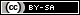This webpage is licensed under a Creative Commons Attribution-ShareAlike License.